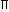# Aptitude - Volume and Surface Area

Exercise : Volume and Surface Area - Data Sufficiency 1
Directions to Solve

Each of the questions given below consists of a statement and / or a question and two statements numbered I and II given below it. You have to decide whether the data provided in the statement(s) is / are sufficient to answer the given question. Read the both statements and

• Give answer (A) if the data in Statement I alone are sufficient to answer the question, while the data in Statement II alone are not sufficient to answer the question.
• Give answer (B) if the data in Statement II alone are sufficient to answer the question, while the data in Statement I alone are not sufficient to answer the question.
• Give answer (C) if the data either in Statement I or in Statement II alone are sufficient to answer the question.
• Give answer (D) if the data even in both Statements I and II together are not sufficient to answer the question.
• Give answer(E) if the data in both Statements I and II together are necessary to answer the question.

1.
 What is the volume of 32 metre high cylindrical tank? I. The area of its base is 154 m2. II. The diameter of the base is 14 m.
I alone sufficient while II alone not sufficient to answer
II alone sufficient while I alone not sufficient to answer
Either I or II alone sufficient to answer
Both I and II are not sufficient to answer
Both I and II are necessary to answer
Explanation:

Given, height = 32 m.

I gives, area of the base = 154 m2.Volume = (Area of the base x Height) = (154 x 32) m3.

Thus, I alone gives the answer.

II gives, radius of the base = 7 m.Volume =r2h =22 x 7 x 7 x 32m3 = 4928 m3. 7

Thus, II alone gives the answer.Correct answer is (C).

2.
 Is a given rectangular block, a cube? I. At least 2 faces of the rectangular block are squares. II. The volume of the block is 64.
I alone sufficient while II alone not sufficient to answer
II alone sufficient while I alone not sufficient to answer
Either I or II alone sufficient to answer
Both I and II are not sufficient to answer
Both I and II are necessary to answer
Explanation:

I gives, any two of l, b, h are equal.

II gives, lbh = 64.

From I and II, the values of l, b, h may be (1 ,1 , 64), (2 ,2 ,16), (4, 4, 4).

Thus, the block may be a cube or cuboid.Correct answer is (D).

3.
 What is the capacity of a cylindrical tank? I. Radius of the base is half of its height which is 28 metres. II. Area of the base is 616 sq. metres and its height is 28 metres.
I alone sufficient while II alone not sufficient to answer
II alone sufficient while I alone not sufficient to answer
Either I or II alone sufficient to answer
Both I and II are not sufficient to answer
Both I and II are necessary to answer
Explanation:

I gives, h = 28 m and r = 14.Capacity =r2h, which can be obtained.

Thus, I alone gives the answer.

II gives,r2 = 616 m2 and h = 28 m.Capacity = (r2 x h) = (616 x 28) m3.

Thus, II alone gives the answer.Correct answer is (C).

4.
 What is the height of a circular cone? I. The area of that cone is equal to the area of a rectangle whose length is 33 cm. II. The area of the base of that cone is 154 sq. cm.
I alone sufficient while II alone not sufficient to answer
II alone sufficient while I alone not sufficient to answer
Either I or II alone sufficient to answer
Both I and II are not sufficient to answer
Both I and II are necessary to answer
Explanation:

II gives the value of r.

But, in I, the breadth of rectangle is not given.

So, we cannot find the surface area of the cone.

Hence, the height of the cone cannot be determined.Correct answer is (D).

5.
 What is the volume of a cube? I. The area of each face of the cube is 64 square metres. II. The length of one side of the cube is 8 metres.
I alone sufficient while II alone not sufficient to answer
II alone sufficient while I alone not sufficient to answer
Either I or II alone sufficient to answer
Both I and II are not sufficient to answer
Both I and II are necessary to answer
I. a2 = 64a = 8 mVolume = (8 x 8 x 8) m3 = 512 m3.
II. a = 8 mVolume = (8 x 8 x 8) m3 = 512 m3.Correct answer is (C).